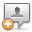KB Home | Advanced Search | News | Ask Question ProSoft Knowledge BaseHome » Categories » Multiple Categories

# Do ProSoft AFC flow computer solutions support energy calculations according to the ISO-6976 standards?

ProSoft AFC flow computer modules implement the Bernoulli equation for measurement of fluid flow through concentric square-edged orifices. The Coefficient of Discharge in this equation is calculated by an appropriate Standard, which may, by option selection, be chosen as either AGA 3 (1992) or ISO-5167 (2003). For energy measurement (heating value, calorific content), the AFC currently uses the equations and values described and specified in Appendix C.4 of AGA 8 (1992), calculating either gross (superior) or net (inferior) ideal energy content (gross/net selected by option) corrected to the user's chosen reference temperature.

As for how these standards compare with the specifications of the ISO-6976 Standard, please consider the following:

1. Energy (heating value, calorific content): As stated before, the AFC Flow Computers use the methods and values given in AGA 8 (1992) Appendix C.4 to calculate molar heating value. This calculation is fundamentally identical to that of ISO-6976, with these caveats:

(a) There is some minor variation between the two Standards in some of the numerical values, specifically the pure-component enthalpies at 25°C. Such numerical properties of pure components must be determined by careful measurement and are expected to be revised from time to time as newer experimental results are accepted as Standard.

(b) ISO recommends selecting the pure-component enthalpies from the tabulated column that corresponds to the user's selected reference temperature, but states that these values were calculated from the 25°C values using one or another method (references given), often involving a polynomial expression of Cp (specific heat at constant pressure) as a function of temperature. ISO's reason for giving tabulated values instead of computation procedure is to simplify the calculations that the user must perform and avoid the need for machine computation (programmed computer). On the other hand, AGA 8 lists the coefficients of a second-degree (order 2, quadratic) polynomial for Cp over temperature, and the ProSoft AFC Flow Computers implement the programmed-computer method We do not yet know which, if any, of the ISO calculation references the values in AGA 8 are derived from, but ISO states that there is no significant difference among any of their several referenced methods over the temperature range 0°C to 25°C, which is the range of reference temperature permitted by the ProSoft AFC Flow Computers. The advantage of AGA 8's method is that the user can choose any reference temperature between 0°C and 25°C instead of being limited to a choice among four specific values (though these four values cover the vast majority of all choices for metric reference temperature, and an appendix gives the enthalpies for the fifth temperature of 60°F.)

(c) Besides heating value on a molar basis, the AFC also calculates it on both a mass and a volume basis. All three values are characteristic of the fluid, depending only on component analysis and reference temperature (volume depends also on reference pressure); calculations thereof are identical to those specified by ISO (other than units conversion), except for the real-gas density used for the volume calculation (see 2 next). Energy accumulations and flow rates are calculated by multiplying the appropriate characteristic value with the increment or flow rate of the primary input (mass or volume) scaled as necessary for units of representation.

2. Density and Compressibility (compression factor):

These values are calculated according to AGA 8 (1992) Detail Characterization Method, and not according to the much simpler method of the ISO Standard (which seems to be very similar to that of pre-1992 AGA 8). ISO's rationale for using the older method is to "enable tractable calculations to be made without necessitating machine computation" One would expect therefore that greater accuracy can be obtained by using AGA 8, and this expectation is supported by the fact that the compressibilities tabulated in ISO-6976 are to only 4 digits for the major components of natural gas and to 3 digits for the less common components; the "summation factors" used in the ISO calculation are derived from those values, so the uncertainty in the resulting compressibility will be relatively high regardless of accuracy. Furthermore, ISO lists values only for three common metric reference temperatures (excluding even 60°F) while AGA 8 permits computation for any reasonable reference temperature, as described in 1(b) above. The only clear advantage of ISO-6976 over AGA 8 is the greatly expanded list of pure components.

3. Wobbe index:

This is not calculated by ProSoft AFC flow computers.
Custom Fields
Attachments (0)Comments